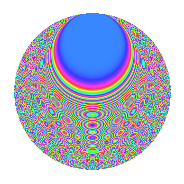# Properties

 Label 3150.2.uLevel 3150 Weight 2 Character orbit u Rep. character $$\chi_{3150}(551,\cdot)$$ Character field $$\Q(\zeta_{6})$$ Dimension 304 Sturm bound 1440

# Learn more about

## Defining parameters

 Level: $$N$$ = $$3150 = 2 \cdot 3^{2} \cdot 5^{2} \cdot 7$$ Weight: $$k$$ = $$2$$ Character orbit: $$[\chi]$$ = 3150.u (of order $$6$$ and degree $$2$$) Character conductor: $$\operatorname{cond}(\chi)$$ = $$63$$ Character field: $$\Q(\zeta_{6})$$ Sturm bound: $$1440$$

## Dimensions

The following table gives the dimensions of various subspaces of $$M_{2}(3150, [\chi])$$.

Total New Old
Modular forms 1488 304 1184
Cusp forms 1392 304 1088
Eisenstein series 96 0 96

## Trace form

 $$304q$$ $$\mathstrut +\mathstrut 152q^{4}$$ $$\mathstrut +\mathstrut 2q^{7}$$ $$\mathstrut +\mathstrut 6q^{9}$$ $$\mathstrut +\mathstrut O(q^{10})$$ $$304q$$ $$\mathstrut +\mathstrut 152q^{4}$$ $$\mathstrut +\mathstrut 2q^{7}$$ $$\mathstrut +\mathstrut 6q^{9}$$ $$\mathstrut -\mathstrut 6q^{13}$$ $$\mathstrut +\mathstrut 6q^{14}$$ $$\mathstrut -\mathstrut 152q^{16}$$ $$\mathstrut -\mathstrut 18q^{17}$$ $$\mathstrut -\mathstrut 4q^{18}$$ $$\mathstrut -\mathstrut 2q^{21}$$ $$\mathstrut +\mathstrut 6q^{24}$$ $$\mathstrut -\mathstrut 12q^{26}$$ $$\mathstrut -\mathstrut 2q^{28}$$ $$\mathstrut -\mathstrut 6q^{29}$$ $$\mathstrut +\mathstrut 6q^{31}$$ $$\mathstrut -\mathstrut 42q^{33}$$ $$\mathstrut -\mathstrut 2q^{37}$$ $$\mathstrut +\mathstrut 6q^{39}$$ $$\mathstrut -\mathstrut 6q^{41}$$ $$\mathstrut +\mathstrut 2q^{42}$$ $$\mathstrut -\mathstrut 2q^{43}$$ $$\mathstrut -\mathstrut 12q^{44}$$ $$\mathstrut -\mathstrut 6q^{46}$$ $$\mathstrut -\mathstrut 18q^{47}$$ $$\mathstrut -\mathstrut 14q^{49}$$ $$\mathstrut -\mathstrut 4q^{51}$$ $$\mathstrut +\mathstrut 36q^{53}$$ $$\mathstrut +\mathstrut 18q^{54}$$ $$\mathstrut +\mathstrut 6q^{57}$$ $$\mathstrut +\mathstrut 12q^{58}$$ $$\mathstrut +\mathstrut 30q^{59}$$ $$\mathstrut -\mathstrut 24q^{61}$$ $$\mathstrut +\mathstrut 36q^{62}$$ $$\mathstrut +\mathstrut 38q^{63}$$ $$\mathstrut -\mathstrut 304q^{64}$$ $$\mathstrut +\mathstrut 14q^{67}$$ $$\mathstrut -\mathstrut 36q^{68}$$ $$\mathstrut -\mathstrut 42q^{69}$$ $$\mathstrut -\mathstrut 8q^{72}$$ $$\mathstrut +\mathstrut 30q^{77}$$ $$\mathstrut -\mathstrut 16q^{78}$$ $$\mathstrut +\mathstrut 20q^{79}$$ $$\mathstrut -\mathstrut 14q^{81}$$ $$\mathstrut +\mathstrut 2q^{84}$$ $$\mathstrut -\mathstrut 48q^{87}$$ $$\mathstrut -\mathstrut 24q^{89}$$ $$\mathstrut -\mathstrut 12q^{91}$$ $$\mathstrut +\mathstrut 30q^{92}$$ $$\mathstrut -\mathstrut 38q^{93}$$ $$\mathstrut +\mathstrut 6q^{96}$$ $$\mathstrut -\mathstrut 6q^{97}$$ $$\mathstrut +\mathstrut 24q^{98}$$ $$\mathstrut +\mathstrut 10q^{99}$$ $$\mathstrut +\mathstrut O(q^{100})$$

## Decomposition of $$S_{2}^{\mathrm{new}}(3150, [\chi])$$ into irreducible Hecke orbits

The newforms in this space have not yet been added to the LMFDB.

## Decomposition of $$S_{2}^{\mathrm{old}}(3150, [\chi])$$ into lower level spaces

$$S_{2}^{\mathrm{old}}(3150, [\chi]) \cong$$ $$S_{2}^{\mathrm{new}}(63, [\chi])$$$$^{\oplus 6}$$$$\oplus$$$$S_{2}^{\mathrm{new}}(126, [\chi])$$$$^{\oplus 3}$$$$\oplus$$$$S_{2}^{\mathrm{new}}(315, [\chi])$$$$^{\oplus 4}$$$$\oplus$$$$S_{2}^{\mathrm{new}}(630, [\chi])$$$$^{\oplus 2}$$$$\oplus$$$$S_{2}^{\mathrm{new}}(1575, [\chi])$$$$^{\oplus 2}$$